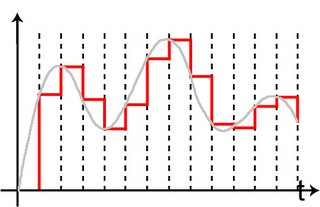Wednesday, November 15, 2006

Choosing the DAC, Part 1 -- Basic Theory

When we talk about digital to analog conversion in our application, we are referring to converting PCM (pulse-code modulation) data into an analog signal. This resulting analog signal can either be represented as an electrical voltage or current. So, for a voltage output, a number is the input and a voltage is the output. These numbers are updated at the sampling interval (or sampling rate) of x times per second. For example, CD data is represented with a 16-bit number at a sampling rate of 44,100 times per second. So a 16-bit number, which represents the amplitude of the audio at a point in time is sent to the DAC (digital to analog converter), along with a clock signal, which causes each number to be stored with the correct sequence and timing (lets ignore that relatively important detail for now). Then the output voltage changes very rapidly from the previous value to the value represented by the currently stored number. So basically, the voltage (or current) is set and held in time until the next number is set. What you end up with is a bunch of rectangular pulses that look like the red line shown in the graph below, instead of the smooth gray lines which represent the ideal analog signal.The differences between the red stair steps and the smooth waveform create a type of distortion in the signal called aliasing. According to good ole Harry Nyquist, the aliasing distortion can be avoided if the sampling frequency is two times greater than the bandwidth of the maximum frequency of your signal. That may not make a lot of sense, so let me use CD audio in another example. Many experts believe the frequency range of human hearing is between 20 and 20,000 Hz. For the majority of us, we can’t hear any sounds above or below that range. So, if you apply the Nyquist sampling theorem (actually Vladimir Kotelnikov and Claude Shannon deserve some mention here, but our friend Harry seems to get all the credit) and double the upper range, we get a sampling rate of 40,000 Hz. So to avoid the aliasing distortion, 44,100 Hz was selected as the sampling frequency for audio CDs.